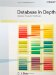# Exercises

##### Exercise 2-1

What's a type? What's the difference between a domain and a type?

##### Exercise 2-2

What do you understand by the term selector?

##### Exercise 2-3

What's a THE_ operator?

##### Exercise 2-4

Physical representations are always hidden from the user: true or false?

##### Exercise 2-5

Elaborate on the following: argument versus parameter; database versus DBMS; generated type versus nongenerated type; scalar versus nonscalar; type versus representation; user-defined type versus system-defined type; user-defined operator versus system-defined operator.

##### Exercise 2-6

What do you understand by the term coercion? Why is coercion a bad idea?

##### Exercise 2-7

Why doesn't "domain check override" make sense?

##### Exercise 2-8

What's a type generator?

##### Exercise 2-9

Define first normal form.

##### Exercise 2-10

Let X be an expression. What's the type of X? What's the significance of the fact that X is of some type?

##### Exercise 2-11

Using the definition of the REFLECT operator in the body of the chapter as a pattern, define a Tutorial D operator that, given an integer, returns the cube of that integer.

##### Exercise 2-12

Use Tutorial D to define an operator that, given a point with cartesian coordinates x and y, returns the point with cartesian coordinates f(x) and g(y), where f and g are predefined operators.

##### Exercise 2-13

Give an example of a relation type. Distinguish between relation types, relation values, and relation variables.

##### Exercise 2-14

Use SQL or Tutorial D (or both) to define relvars P and SP from the suppliers-and-parts database. If you give both SQL and Tutorial D definitions, identify as many differences between them as you can. What's the significance of the fact that relvar P (for example) is of a certain relation type?

##### Exercise 2-15

Given the types specified in the introductory section for attributes in the suppliers-and-parts database, which of the following scalar expressions are valid? For those that are, state the type of the result; for the others, show an expression that will achieve what appears to be the desired effect.

` a. CITY = 'London' b. SNAME || PNAME c. QTY * 100 d. QTY + 100 e. STATUS + 5 f. 'ABC' < CITY g. COLOR = CITY h. CITY || 'burg'`

##### Exercise 2-16

It's sometimes suggested that types are really variables, in a sense. For example, employee numbers might grow from three digits to four as a business expands, so we might need to update "the set of all possible employee numbers." Discuss.

##### Exercise 2-17

A type is a set of values, and the empty set is a legitimate set; thus, we might define an empty type to be a type where the set in question is empty. Can you think of any uses for such a type?

##### Exercise 2-18

In the body of the chapter, I said the equality comparison operator "=" applies to every type (though I didn't spell out the semantics, which are that if v1 and v2 are values of the same type, then v1 = v2 evaluates to TRUE if and only if v1 and v2 are the very same value). As explained in more detail in Chapter 8, however, SQL doesn't require "=" to apply to every type, nor does it prescribe the semantics in all of the cases where it does apply. What are the implications of this state of affairs?

##### Exercise 2-19

Following on from the previous exercise, we can say that v1 = v2 evaluates to TRUE if and only if executing some operator Op on v1 and executing that same operator Op on v2 always has exactly the same effect, for all possible operators Op. But this is another precept that SQL violates. Can you think of any examples of such violation? What are the implications?

##### Exercise 2-20

Why are pointers excluded from the relational model?

##### Exercise 2-21

The Assignment Principle which is very simple, but fundamental states that after assignment of the value v to the variable V, the comparison V = v evaluates to TRUE. Yet again, however, this is a precept that SQL violates (fairly ubiquitously, in fact). Can you think of any examples of such violation? What are the implications?

##### Exercise 2-22

Do you think that types "belong to" databases, in the same sense that relvars do?

##### Exercise 2-23

In the section "Domain-Constrained Comparisons," I showed an SQL SELECT - FROM - WHERE expression that contained another such expression nested inside it (a "subquery"). Now, each of the SELECT clauses in the overall expression could have been replaced by the arguably simpler form SELECT* ("SELECT star"). But "SELECT *" suffers from certain problems, which is why I usually not invariably avoid using it in this book. Identify as many of those problems as you can. Can you think of any other constructs in SQL that suffer from similar problems?

##### Exercise 2-24

In the first example of an SQL SELECT expression in this chapter, I pointed out that there was no terminating semicolon because the SELECT expression was an expression and not a statement. But what's the difference?Database in Depth: Relational Theory for Practitioners
ISBN: 0596100124
EAN: 2147483647
Year: 2006
Pages: 127
Authors: C.J. Date

Similar book on Amazon